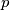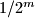IMO Shortlist 2013 problem C8

Kvaliteta:
Avg: 0,0
Težina:
Avg: 9,0
Players$A$ and$B$ play a paintful game on the real line. Player$A$ has a pot of paint with four units of black ink. A quantity$p$ of this ink suffices to blacken a (closed) real interval of length$p$. In every round, player$A$ picks some positive integer$m$ and provides$1/2^m$ units of ink from the pot. Player$B$ then picks an integer$k$ and blackens the interval from$k/2^m$ to$(k+1)/2^m$ (some parts of this interval may have been blackened before). The goal of player$A$ is to reach a situation where the pot is empty and the interval$[0, 1]$ is not completely blackened.

Decide whether there exists a strategy for player$A$ to win in a finite number of moves.
Izvor: Austria